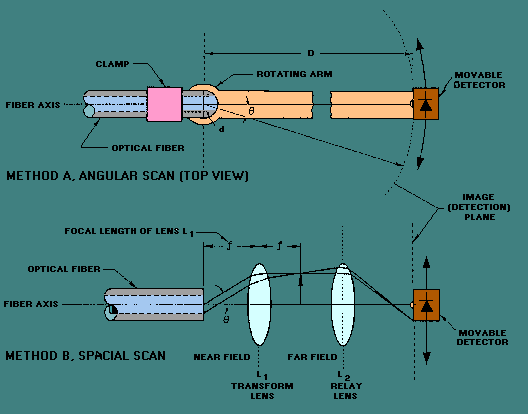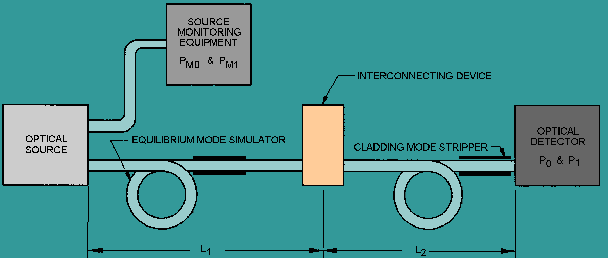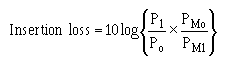radiation pattern. Our discussion is limited to measuring the NA from the output far-field radiation pattern. The NA of a multimode fiber having a near-parabolic refractive index profile is measured using EIA/TIA-455-177. "> Numerical ApertureCustom SearchNumerical Aperture The numerical aperture (NA) is a measurement of the ability of an optical fiber to capture light. The NA can be defined from the refractive index profile or the output far-field radiation pattern. Our discussion is limited to measuring the NA from the output far-field radiation pattern. The NA of a multimode fiber having a near-parabolic refractive index profile is measured using EIA/TIA-455-177. In EIA/TIA-455-177, the fiber NA is measured from the output far-field radiation pattern. The far-field power distribution describes the emitted power per unit area in the far-field region. The far-field region is the region far from the fiber-end face. The far-field power distribution describes the emitted power per unit area as a function of angle Θ some distance away from the fiber-end face. The distance between the fiber-end face and detector in the far-field region is in the centimeters (cm) range for multimode fibers and millimeters (mm) range for single mode fibers. EIA/TIA-455-47 describes various procedures, or methods, for measuring the far-field power distribution of optical waveguides. These procedures involve either an angular or spacial scan. Figure 5-10 illustrates an angular and spacial scan for measuring the far-field power distribution. Figure 5-10 - Angular and spacial scan methods for measuring the far-field power distribution.Figure 5-10 (method A) illustrates a far-field angular scan of the fiber-end face by a rotating detector. The fiber output radiation pattern is scanned by a rotating detector in the far-field. The detector rotates in a spherical manner. A record of the far-field power distribution is kept as a function of angle Θ. Figure 5-10 (method B) illustrates a far-field spacial scan of the fiber-end face by a movable (planar) detector. In a far-field spacial scan, lens L1 performs a Fourier transform of the fiber output near-field pattern. A second lens, L2, is positioned to magnify and relay the transformed image to the detector plane. The image is scanned in a plane by a movable detector. The scan position  y in the Fourier transform plane is proportional to the far-field scan angle Θ. A record of the far-field power distribution is kept as a function of the far-field scan angle. The normalized far-field pattern is plotted as a function of the far-field scan angle Θ. Figure 5-11 shows the plot of the normalized far-field radiation pattern as a function of scan angle. Figure 5-11. - Normalized far-field radiation pattern.Fiber NA is defined by the 5 percent intensity level, or the 0.05 intensity level, as indicated in figure 5-11. The 0.05 intensity level intersects the normalized curve at scan angles ΘA and ΘB. The fiber NA is defined aswhere Θ5 is the 5 percent intensity half angle. Θ5 is determined from ΘA and ΘB as shown below:Q.19 Far-field power distributions describe the emitted power per unit area as a function of angle Θ in the far-field region. Describe the far-field region. Mode Field Diameter The mode field diameter (MFD) of a single mode fiber is related to the spot size of the fundamental mode. This spot has a mode field radius w0. The mode field diameter is equal to 2w0. The size of the mode field diameter correlates to the performance of the single mode fiber. Single mode fibers with large mode field diameters are more sensitive to fiber bending. Single mode fibers with small mode field diameters show higher coupling losses at connections. The mode field diameter of a single mode fiber can be measured using EIA/TIA-455-167. This method involves measuring the output far-field power distribution of the single mode fiber using a set of apertures of various sizes. This far-field power distribution data is transformed into the near-field before using complex mathematical procedures. The mode field diameter is calculated from the transformed near field data. The mathematics behind the transformation between the far-field and near-field is too complicated for discussion in this chapter. Refer to EIA/TIA-455-167 for information on this transformation procedure. Q.20 Will fiber coupling loss generally increase or decrease if the mode field diameter of a single mode fiber is decreased?   Insertion Loss Insertion loss is composed of the connection coupling loss and additional fiber losses in the fiber following the connection. In multimode fiber, fiber joints can increase fiber attenuation following the joint by disturbing the fiber's mode power distribution (MPD). Fiber joints may increase fiber attenuation because disturbing the MPD may excite radiative modes. Radiative modes are unbound modes that radiate out of the fiber contributing to joint loss. In single mode fibers, fiber joints can cause the second-order mode to propagate in the fiber following the joint. As long as the coupling loss of the connection is small, neither radiative modes (multimode fiber) or the second-order mode (single mode fiber) are excited. Insertion loss of both multimode and single mode interconnection devices is measured using EIA/TIA-455-34. For Navy applications, an overfill launch condition is used at the input fiber. For other applications a mandrel wrap may be used to strip out high-order mode power. The length of fiber before the connection and after the connection may be specified for some applications. Power measurements are made on an optical fiber or fiber optic cable before the joint is inserted and after the joint is made. Figure 5-12 illustrates the mandrel wrap method of measuring the insertion loss of an interconnecting device in EIA/TIA-455-34. Figure 5-12. - Insertion loss measurement of an interconnecting device.Initial power measurements at the detector (P0) and at the source monitoring equipment (PM0) are taken before inserting the interconnecting device into the test setup. The test fiber is then cut at the location specified by the end user. The cut results in a fiber of lengths L1 and L2 before and after the interconnection device that simulates the actual system configuration. After interconnection, the power at the detector (P1) and at the source monitoring equipment (PM1) is measured. The insertion loss is calculated as shown below:If the source power is constant, then the calculation of the insertion loss is similar to that of fiber attenuation. Q.21 In multimode fibers, how do fiber joints increase fiber attenuation following the joint?Integrated Publishing, Inc. - A (SDVOSB) Service Disabled Veteran Owned Small Business